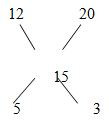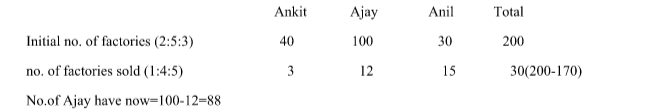# RRB ALP 2018 Practice Test Papers | Arithmetic Questions (Day-9)

Dear Aspirants, Here we have given the Important RRB ALP & Technicians Exam 2018 Practice Test Papers. Candidates those who are preparing for RRB ALP 2018 can practice these Arithmetic Questions to get more confidence to Crack RRB 2018 Examination.

[WpProQuiz 1532]

Click “Start Quiz” to attend these Questions and view Explanation

1. A mixture of 50 l of water and milk contains 75% milk. How many litres of water to be added to make the concentration of milk to 50%?
1. 50
2. 12.5
3. 25
4. 37.5
1. Harini covered 150 km in 10 hours. She covered part of his journey by car an another part by scooter. The speed of car and scooter is 20 km/hr and 12 km/hr respectively. What is the ratio of the distances covered by car and scooter?
1. 2:3
2. 1:1
3. 4:5
4. none of the above
1. The ratio of acid and mixture of acid and distilled water is 1:5. how many litres of distilled water to be replaced  to make the concentration of acid to 30% if initially  there was 32 litres of distilled water?
1. 4
2. 8
3. 12
4. 15
1. Ankit, Ajay and Anil have factories in the ratio 2:5:3. Due to loss in business they sold some of their factories in the ratio 1:4:5 respectively. What is the number of factories Ajay have now if the initially they have 200 factories in total and 170 factoriesnow?
1. 88
2. 56
3. 78
4. 48
1. In a coffee shop there is a offer on valentine’s day i.e. 15% discount on total rate for couple. Mr.& Mrs. Raj ordered cold coffee which costs 200 each and Mr. & Mrs. Harish ordered Espresso which costs 150 each. What is the bill amount for the above order.
1. 515
2. 297.5
3. 395
4. 595
1. The average of 10, 20, 30,40 ….100 is 55. If each number is multiplied by 2 and then subtracted by 5 what is the new average?
1. 95
2. 100
3. 105
4. 110
1. The average age of 20 English professors in a college is 44 years, the number of Maths Professors is three fourth of the number of English professors then what is the average age of Maths professors?
1. 88
2. 33
3. none of these
4. cannot be determined
1. Brinda travels from Gurgaon to Agartala at the speed of 40 km/hr and returns from Agartala to gurgaon at the speed of x km/hr. Find the average speed of Brinda if the ratio of sum of two speeds ie to and fro and difference of two speeds is 5:1.(x>40)
1. 48 km/hr
2. 50 km/hr
3. 40 km/hr
4. 32 km/hr
1. The cost of 3 BHK flat is three fourth of difference between between 1 BHK flat and a villa. The cost of 1 BHK flat and villa is 40 lakhs and 1.4crore respectively and the cost of 2 BHK flat is two third of the cost of 3 BHK flat. What is the sum of the costs of 2 BHK flat and 1 BHK flat?
1. 80 lakh
2. 75 lakh
3. 90 lakh
4. 1 crore
1. 5 years ago the average age of family of 5 members is 32 years. Krish got married today and the average increases by 3. What is the age of Mrs. Krish?
1. 35
2. 30
3. 25
4. cannot be determined

Explanation:

Quantity of milk and water in 50 l= milk-37.5 and water 12.5

To make concentration of milk to 50%

12.5+x= 50/100(50+x)

X=25

Average speed of Harini= 150/10= 15 km/hrRatio of time taken of car to scooter is 5:3

Ratio of distance covered is 5*12: 3*20= 1:1

Ratio of acid to water is 1:4

Quantity of acid=32/4*1=8

Quantity of water to be replaced= (30/100*40)-8=4No.of Ajay have now=100-12=88

15/100(200*2)+15/100(150*2)=595

55*2-5=105

40+x/(x-40)=5/2

x=60

average speed= 2*40*60/(40+60)=48

Cost of 3BHK flat = 3/4(1.4 c-40 lakh)=75 lakh

Cost of 2 BHK flat =2/3(75 lakh)=50 lakh

Cost of 2 BHK flat and 1 BHK flat= 50+40=90 lakh

Present average of five members=32+5=37

Sum of ages of five members= 37*5=185

Age of Mrs. Krish =>(185+x)/6=35

Age of Mrs. Krish=25

RRB ALP 2018 Practice Test Papers | Arithmetic Questions (Day-1)

RRB ALP 2018 Practice Test Papers | Arithmetic Questions (Day-2)

RRB ALP 2018 Practice Test Papers | Arithmetic Questions (Day-3)

RRB ALP 2018 Practice Test Papers | Arithmetic Questions (Day-4)

RRB ALP 2018 Practice Test Papers | Arithmetic Questions (Day-5)

RRB ALP 2018 Practice Test Papers | Arithmetic Questions (Day-6)

RRB ALP 2018 Practice Test Papers | Arithmetic Questions (Day-7)

RRB ALP 2018 Practice Test Papers | Arithmetic Questions (Day-8)